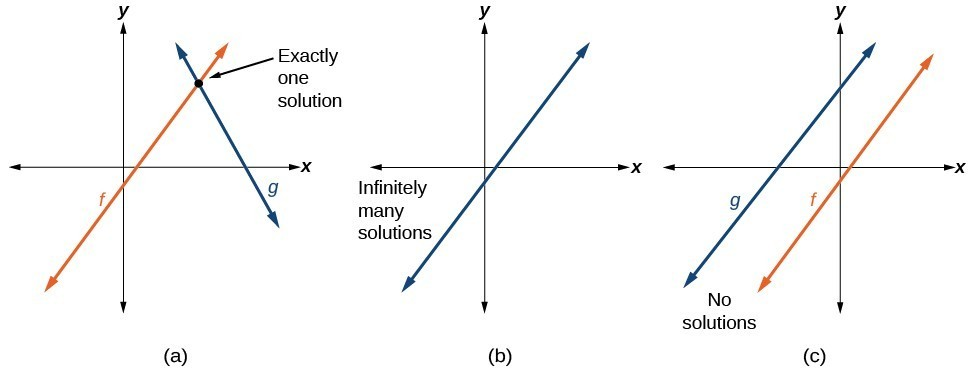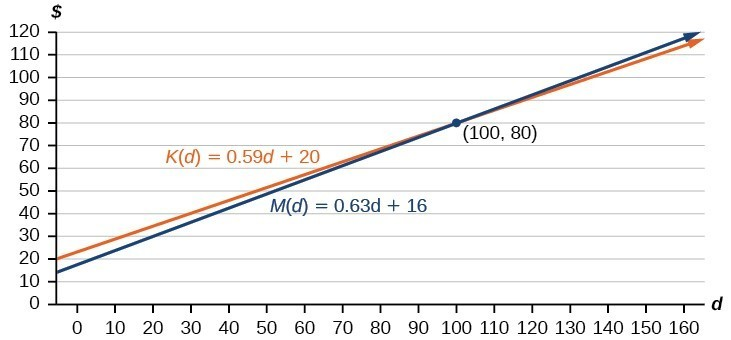## Build systems of linear modelsFigure 6

Real-world situations including two or more linear functions may be modeled with a system of linear equations. Remember, when solving a system of linear equations, we are looking for points the two lines have in common. Typically, there are three types of answers possible.

### How To: Given a situation that represents a system of linear equations, write the system of equations and identify the solution.

1. Identify the input and output of each linear model.
2. Identify the slope and y-intercept of each linear model.
3. Find the solution by setting the two linear functions equal to another and solving for x, or find the point of intersection on a graph.

### Example 4: Building a System of Linear Models to Choose a Truck Rental Company

Jamal is choosing between two truck-rental companies. The first, Keep on Trucking, Inc., charges an up-front fee of $20, then 59 cents a mile. The second, Move It Your Way, charges an up-front fee of$16, then 63 cents a mile1. When will Keep on Trucking, Inc. be the better choice for Jamal?

### Solution

The two important quantities in this problem are the cost and the number of miles driven. Because we have two companies to consider, we will define two functions.

 Input d, distance driven in miles Outputs K(d): cost, in dollars, for renting from Keep on TruckingM(d) cost, in dollars, for renting from Move It Your Way Initial Value Up-front fee: K(0) = 20 and M(0) = 16 Rate of Change K(d) = $0.59/mile and P(d) =$0.63/mile

A linear function is of the form $f\left(x\right)=mx+b$. Using the rates of change and initial charges, we can write the equations

$\begin{cases}K\left(d\right)=0.59d+20\\ M\left(d\right)=0.63d+16\end{cases}$

Using these equations, we can determine when Keep on Trucking, Inc., will be the better choice. Because all we have to make that decision from is the costs, we are looking for when Move It Your Way, will cost less, or when $K\left(d\right)<M\left(d\right)$. The solution pathway will lead us to find the equations for the two functions, find the intersection, and then see where the $K\left(d\right)$ function is smaller.Figure 7

These graphs are sketched in Figure 7, with K(d) in blue.

To find the intersection, we set the equations equal and solve:

$\begin{cases}K\left(d\right)=M\left(d\right)\hfill \\ 0.59d+20=0.63d+16\hfill \\ 4=0.04d\hfill \\ 100=d\hfill \\ d=100\hfill \end{cases}$

This tells us that the cost from the two companies will be the same if 100 miles are driven. Either by looking at the graph, or noting that $K\left(d\right)$ is growing at a slower rate, we can conclude that Keep on Trucking, Inc. will be the cheaper price when more than 100 miles are driven, that is $d>100$.